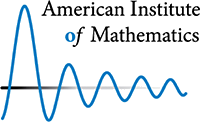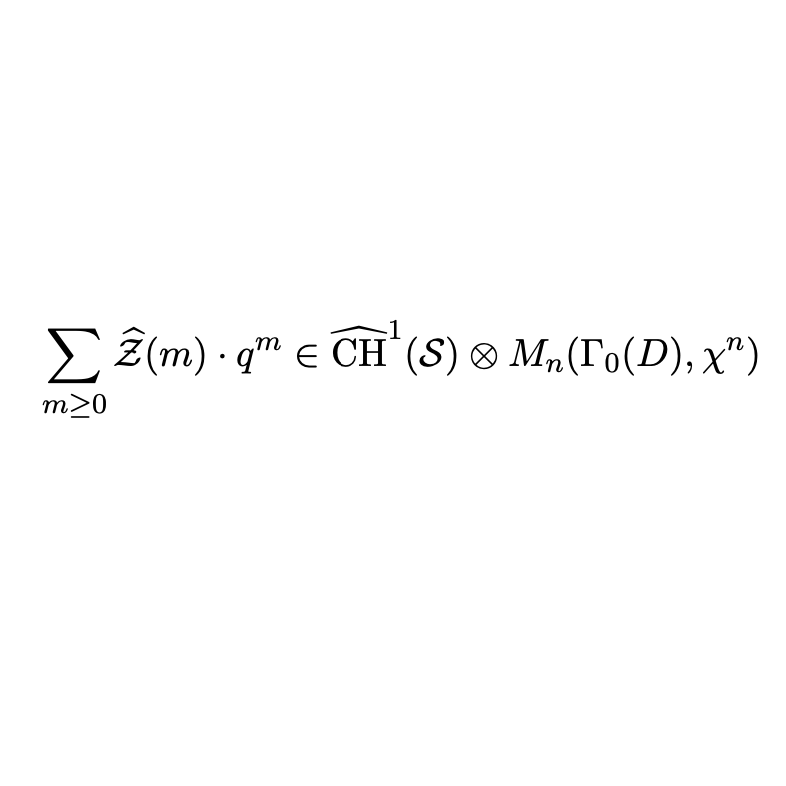### Highlights#### Modularity of Generating Series

AIM - May 2023The formation of generating series is a longstanding technique in many areas of mathematics. Typically, some ordered sequence of numbers is given and the generating series is obtained by taking these quantities as the coefficient of $$q^n$$ in a power series in a parameter $$q$$. For example, if the $$k$$th term in the sequence is the binomial coefficient $$\binom{n}{k}$$, then the series is the familiar
$\sum_{k=0}^n \binom{n}{k} q^k = (1+q)^n.$

If the sequence consists simply of ones, then the series is
$\sum_{k=0}^\infty q^k = \frac{1}{1-q}.$
Notice that in both of these cases, the series collapses to a rational function of $$q$$.

In general, the resulting power series is just a formal object with no analytic meaning. But it can happen that the generating series has a power series expansion that has some special properties related to the theory of modular forms. A modular form is  a function defined on the upper half of the complex plane with some remarkable symmetry properties.

To be more precise, a function $$f$$ is modular when it is analytic in the upper half plane $$(\Im (z) >0)$$, bounded as $$z$$ tends to infinity on the  imaginary axis, and satisfies for all $$a, b, c, d \in \mathbb{Z}, \,\,\mbox{such that} \,\,ad-bc = 1$$
$f\left(\frac{az + b}{cz + d} \right) = (cz +d)^{k}f(z)$
for some $$k$$, usually a positive integer, and all $$z$$ in the upper half plane.

When the generating function coincides with the expansion at infinity of a modular form, the generating series is said to be modular. The main breakthrough of the AIM SQuaRE project was a proof of the modularity of the generating series for some special classes os sequences and resulting in important links between arithmetic geometry and analysis.

For the interested reader, here are more examples and a more technical description of what was done. A noteworthy class of examples of modular generating series are the theta series that arise when the defining sequence $$a(n)$$ is the number of vectors of square length $$2n$$  in an even lattice in Euclidean space. For such series modularity is a consequence of the Poisson summation formula. Since the relevant space of modular forms is known to be finite dimensional, such modularity reflects a very strong coherence among the $$a(n)$$’s and sometimes results in striking identities.

For the root lattice of type $$E_8$$, the relevant space of modular forms has dimension 1 and also contains the Eisenstein series of weight 4, an explicitly constructed modular form of occurring in the theory of elliptic curves. This Eisenstein series can be viewed as the generating series for the numbers $$c(n)$$ given by 240 times the sum of the cubes of the positive divisors of $$n$$, with a suitable term $$c(0) = 1$$ added.  It follows that this Eisenstein series coincides with the $$E_8$$ theta series, proving a striking formula for the number of square length $$2n$$ vectors in $$E_8$$.

Generating series can also be formed from quantities arising in geometry and in arithmetic geometry, in particular from special cycles in unitary Shimura varieties.  Such varieties are constructed as quotients of the complex unit ball of dimension $$m$$ by arithmetic groups coming from Hermitian lattices of signature $$(m,1)$$ over integer rings of imaginary quadratic fields. A rich supply of divisors &mdash; special divisors &mdash; is provided by the images of sub-balls of dimension m-1 that are compatible with the arithmetic group action.  These compatible sub-balls are associated to positive lattice vectors so that one can define special divisors $$Z(n)$$ for positive integers n by collecting lattice vectors of square norm $$2n$$. Generating series are then constructed by taking the classes of the $$Z(n)$$ in suitable cohomology groups. Such cohomology valued generating series can be viewed as analogues of theta series. More generally, one can construct integral models of these unitary Shimura varieties as moduli spaces of abelian schemes with an action of the imaginary quadratic integer ring. The special divisors extend to arithmetic special divisors on such models, defined in terms of moduli.  Now in the formation of generating series the cohomology groups are replaced by Chow groups and arithmetic Chow groups.

The main focus of the AIM SQuaRE project was a proof of the modularity of the generating series for the classes of arithmetic special divisors in the Chow group of a compactified integral model of a unitary Shimura variety.  An analogous modularity result is also proved for the classes of special divisors equipped with suitable Green functions in the Gillet-Soule arithmetic Chow groups. In both cases, the modular generating series should be viewed as a new type of arithmetic theta series.  These series are expected to have many applications as they provide a bridge between the delicate arithmetic geometry of integral models of unitary Shimura varieties and the powerful analytic machinery of modular forms. For example, they have already been used to prove Gross-Zagier style formulas relating derivatives of L-functions to arithmetic intersection pairing on unitary Shimura varieties and special cases of the Colmez conjecture on the Faltings heights of abelian varieties with complex multiplication. More recently the modularity of the Gillet-Soule Chow group valued series has been used by Wei Zhang to prove his arithmetic fundamental lemma relating intersections of cycles on Rapoport-Zink space to derivatives of orbital integrals. The problem of finding higher codimensional generalizations is an active area of current research.